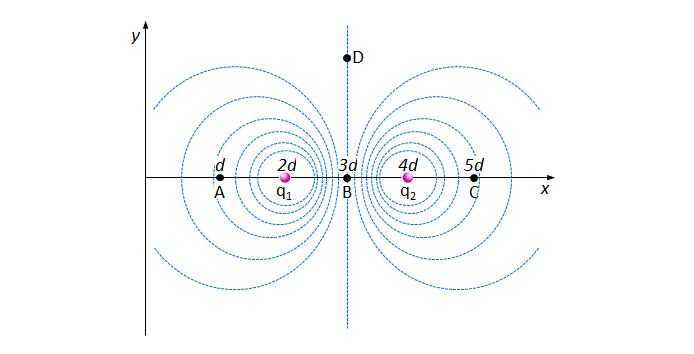# Two charged particlesThe above shows isoelectric lines generated by two charged particles $q_1$ and $q_2$ fixed on the $x$-axis of an $xy$-plane, where $q_1$ is a positively charged particle. The points $A,$ $B$ and $C$ are also on the $x$-axis with the distances from the origin being $d,$ $3d$ and $5d,$ respectively. The isoelectric line passing through the point $B$ is parallel to the $y$-axis, and the point $D$ is on this isoelectric line. Which of the following statements is NOT correct?

a) $q_2$ is a negatively charged particle.

b) The voltage at the point $A$ is higher than the voltage at the point $B$.

c) If we put a positive charge on the point $B$, then it does not move.

d) The direction of the electric field at the point $A$ is the same as that at the point $C$.

×